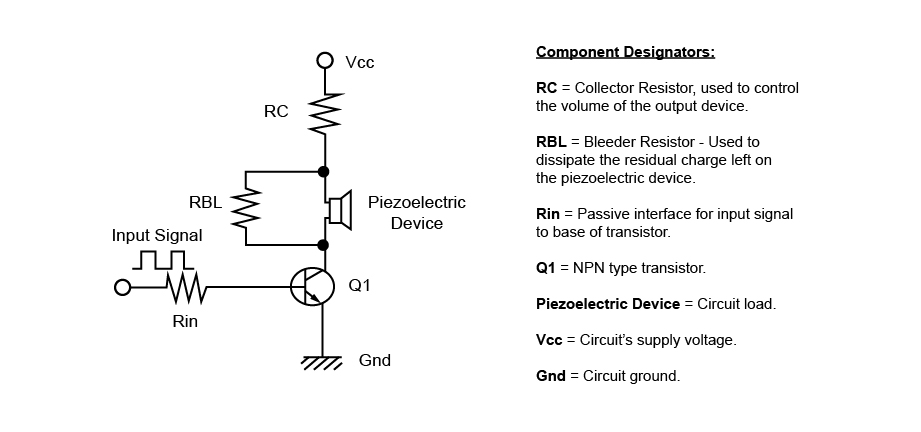# Piezo Ext Drive

## Example of a Drive Circuit for an Externally Driven Piezoelectric Device

A single stage analog amplifier is utilized as an "external drive" circuit for a piezoelectric sounder or a piezoelectric diaphragm device.

In theory, a piezoelectric sounder or a piezoelectric diaphragm will emit "sound" when an AC voltage (signal) is applied to both terminals of the product. If the frequency of the AC voltage (signal) is within the hearing range of 20 Hz - 20 KHz and the amplitude of the AC voltage (signal) is large enough, the human ear will hear the buzzing sound created by the input AC voltage (signal). Below is a simple diagram of the theoretical circuit defined above:Examples of typical drive circuits are broadly divided into two types of driver circuits:

Type 1 is an analog driver circuit. An example is where analog components, such as a transistor and resistive components make up the circuit is used to provide the amplified signal to drive the device.

Type 2 is a digital driver circuit. An example is where a solid state device such as an operational amplifier (digital component) is used to create the drive signal that drives the device.

The circuit (type 1) below is considered a common-emitter amplifier and is known as a "power amplifier" as both the base (input) current and voltage appear to be amplified when compared to the collector (output) portion of the circuit, creating a higher value of power (Voltage x Current) to be applied to the circuit load.

Type 1: Transistor Circuit - Example of a drive circuit for a piezoelectric sounder and a piezoelectric diaphragm (external drive type) in the case where a transistor circuit is used.Key variables in circuit operation:

In order to create a higher volume output from the Piezoelectric Device, you must either increase the circuit's supply Voltage (Vcc) that is provided between circuit ground and Vcc, or increase the input signal's peak to peak value.

The higher the value of the voltage applied to V, the higher the peak-to-peak amplitude signal is presented to create a greater sound pressure of the piezoelectric sound component without circuit signal distortion. Increasing the input signal in will also produce a greater sound pressure of the piezoelectric sound component, but is limited to the circuit's rail values as determined by the Vcc to circuit ground value. Increasing the signal in value to create an output signal greater than the circuit rail values will result in distortion due to the clipping of the peak values of the output signal.

Circuit Notes:

1) The transistor functions as a buffer for protecting the IC from back electromotive force generated by the piezoelectric sound component.

2) Also, in order to protect the transistor itself, consider connecting a Zener diode in parallel with the piezoelectric sound component and RBL if necessary.

3) In the diagram, the piezoelectric sound component and the resistors are connected between the collector of the transistor and V. However, these components can be installed between the emitter of the transistor and GND instead.

4) A FET can be used instead of the transistor.